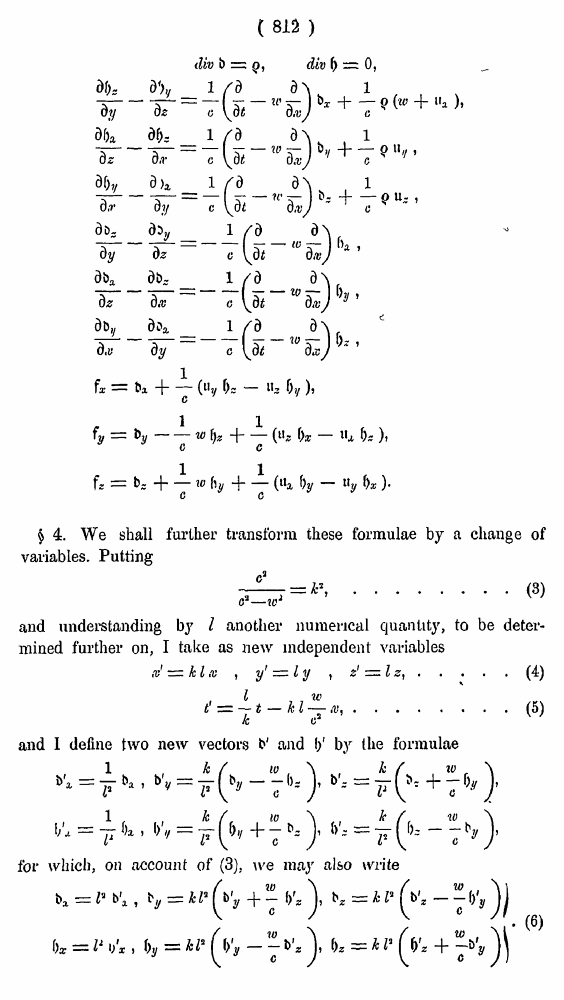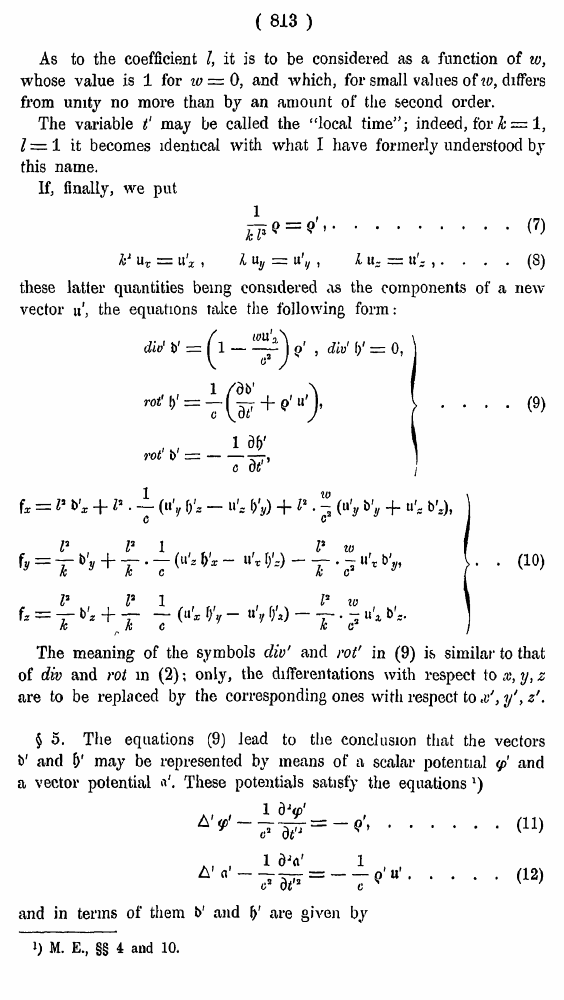# H. A. Lorentz. Electromagnetic phenomena in a system moving with any velocity smaller than that of light. // Proceedings Royal Acad., Amsterdam. Vol. VI., 1904

┬ ĒÓ„ÓļŅ   ─­¾ŃĶÕ ¶Ņ­ņÓ“¹   <<<     č“­ÓĒĶ÷Ó 812   >>>809  810  811  812 813  814  815  816  817  818  819  820  821  822  823  824  825  826  827  828  829  830  831 ( 815 ) \$ 4. We shall further transform these formulae by a change of variables. Putting ........(3) and understanding by I another numerical quantity, to be determined further on, I take as new independent variables .....(4) .....(5) and I define two new vectors b' and I)' by the formulae tor which, on account of (3), we may also write ( 813 ) As to the coefficient I, it is to be considered as a function of w, whose value is 1 for 10 = 0, and which, for small values of to, differs from unity no more than by an amount of the second order. The variable tæ may be called the ōlocal time5Æ; indeed, for h = 1, Z = 1 it becomes identical with what I have formerly understood by this name. If, finally, we put ... (7) ... (8) these latter quantities being considered as the components of a new vector ½', the equations take the following form : . . (9) . (10) The meaning of the symbols div' and rot' in (9) ivS similar to that of div and rot in (2); only, the differentations with respect to x, y, z are to be replaced by the corresponding ones with respect to y', z'. ¦ 5. The equations (9) lead to the conclusion that the vectors b' and I)1 may be represented by means of a scalar potential ' and &' are given by 1) M. E., ¦¦ 4 and 10.